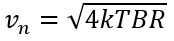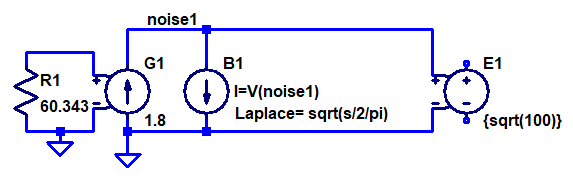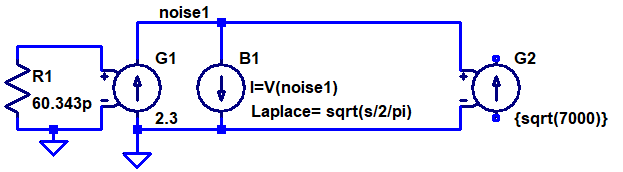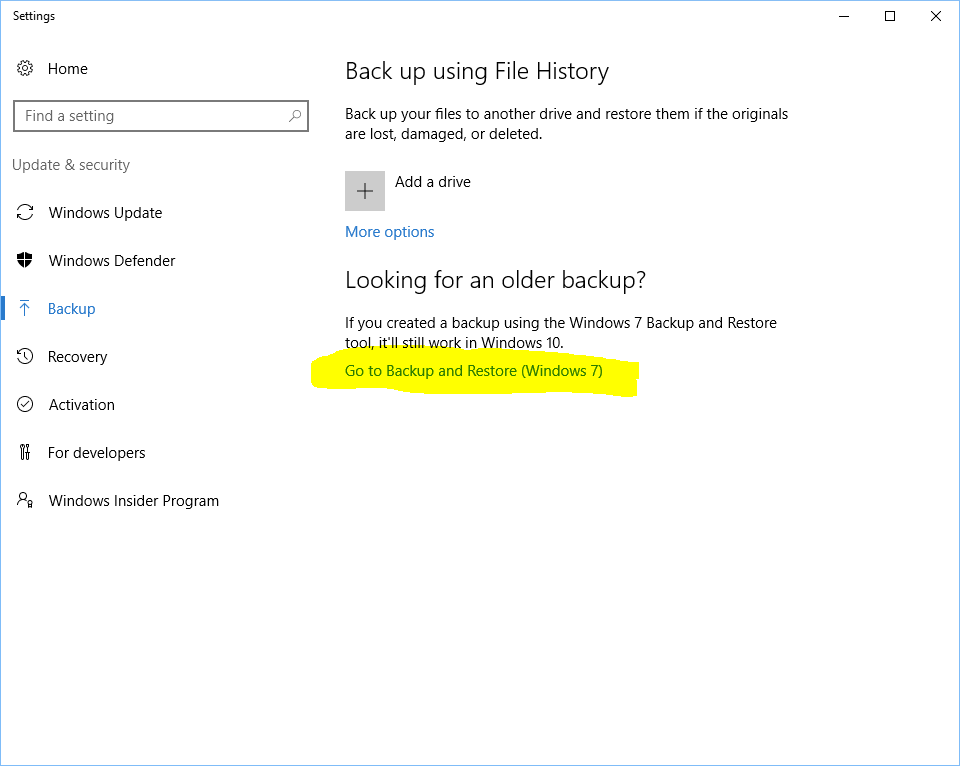# Voltage and Current Noise Sources in LTspice .noise Simulations

There does not seem to be a direct way of adding a voltage noise or current noise source to an LTspice (or other kinds of Spice for that matter) circuit to be used in a .noise simulation. It is however possible to add noise sources to be used in .tran (time domain) simulations using behavioral sources, but this is not what this post is about. Instead it shows a method of adding white (Johnson as well as shot) and 1/f (flicker) voltage or current noise sources of the desired amplitude to be used in .noise simulations.

One case where such noise sources can be useful is when making simulation models of amplifiers (like opamps) where the input referred voltage and current noises are known from the datasheet.

The only simple noise source (that affects .noise simulations) in LTspice is a simple resistor. Other noise sources exist in semiconductor device models, but those models are more complex and messy. An ideal resistor has a voltage noise described by:Where k is Botzmann’s constant (1.381×10-23 J/K), T is the temperature in Kelvin (300 K by default in LTspice), B is the bandwidth in Hz and R is the resistance in Ω.

A datasheet for an amplifier typically specifies the white voltage noise in units of nV/√Hz and current noise in fA/√Hz (sometimes pA/√Hz).

So, can we somehow create noisy voltage and current sources based on noisy resistors? The answer is yes, by using dependent sources. To create a white voltage noise source, we can connect the input terminals of a voltage dependent voltage source (E source) to a resistor and use a suitable scaling factor. The dependent source isolates the resistor from any circuitry that is connected to it and preserves the voltage noise amplitude regardless of load.

As mentioned above, the noise source we are trying to model is usually specified in nV/√Hz, so it would be convenient to be able to directly enter that number as part of the model. A simple way of doing that is to select a resistance that produces a noise density of 1 nV/√Hz and enter the noise amplitude from the datasheet as the voltage gain of the dependent source. Solving the above equation for vn = 1 nV when T = 300 K and B = 1 Hz gives R = 60.343 Ω.

The resulting LTspice schematic for a 4.5 nV/√Hz voltage noise source is thus:

Similarly, to create a white current noise source, we can use a voltage dependent current source (G source). To allow us to set the transconductance factor of the source to the noise density in fA/√Hz, we need a resistor with a noise density of 1 fV/√Hz, which means that the resistor shall have the very small value of 60.343 pΩ (piko ohm)! A current noise source with a noise density of 4 fA/√Hz can thus be modeled like this:

Often, one is also interested in flicker noise, whose power density is proportional to 1/f, i.e. it decreases with frequency. If the power density is proportional to 1/f, the voltage (or current) noise density is proportional to 1/√f. This makes it a bit harder to create a model for this kind of noise in LTspice, but it is still possible. The trick is to use the white current noise source above and connect it to a behavioral current source (B source) which has a Laplace function that makes it behave like an impedance whose magnitude is 1/√f. I found the documentation to be a bit unclear on behavioral sources, but after some experimentation I got the following to work:Flicker voltage noise source with an amplitude of 1.8 nV/√Hz at 100 Hz.

The documentation for a behavioral source in LTspice says that “If an optional Laplace transform is defined, that transform is applied to the result of the behavioral current or voltage.”. It seems that applied in this case means divided by. Unexpected in my opinion.

So the way this B source works is that it produces a current that is the same as the voltage across it (divided by an implicit resistance of 1 Ω to get the units right) and this current is modified by dividing it in the Laplace/frequency domain by the Laplace expression √(s/2π). The complex frequency variable s of the Laplace transform can be written as s = σ+jω = σ+j2πf where f is the frequency in Hz (and σ is the hard-to-interpret real part of s, which can be set to 0 to essentially convert the Laplace transform into a Fourier transform). By dividing s by , we get the desired behavior of an impedance whose magnitude is equal to 1/√f. This impedance is not a pure resistance, but a complex impedance that will cause a phase shift of 45 degrees between current and voltage. Phase shifts are however irrelevant when dealing with noise (unless there are multiple signal paths from a noise source to a node) and the important thing here is that the magnitude of the impedance is right. If we wanted to model a resistor with a resistance of 1/√f, we could divide s by √-1 to make the impedance real, like this: Laplace = sqrt(s/2/pi/sqrt(-1)). This would work equally well in our model for flicker noise, but the expression is bigger and clumsier without changing the noise simulation results.

The level of flicker noise is typically given in one of two ways in datasheets. Either one can read the level off a graph at some frequency where the flicker noise dominates, or it is specified as the corner frequency fc at which it is equal to the white noise level at the same node. In both cases we know the noise level at some frequency and we would of course like to be able to input these two numbers into the noise generator model. This is done by setting the noise level (at the specified frequency) as the transconductance of the G source and by setting the gain of the E source to the square root of the specified frequency. The reason we need to use the square root of the frequency is of course that the white noise current from the G source is multiplied by 1/√f by the B source and that we want to scale it back up to the same intensity precisely at fc. Multiplying the noise by √fc obviously cancels the 1/√f  factor at fc.

A flicker current noise generator can be created in a very similar manner. The only difference is that the output is produced by a G source and that the noise generating resistor is 60.343 pΩ. Here is an example:Flicker current noise source with an amplitude of 2.3 fA/√Hz at 7000 Hz.

So let’s put all of this together and create a noise model of an opamp connected as a voltage follower like this:

As an example, I selected OPA838 whose datasheet contains the following noise specifications:

Here is the resulting noise model with all four noise sources:

Link to the above LTspice schematic

The white current noise is 1000 fA/√Hz (R3, G2) and the current noise corner frequency is 7000 Hz (R4, G3, B2, G4). The white voltage noise is 1.8 nV/√Hz (R2, E2) and the voltage noise corner frequency is 100 Hz (R1, G1, B1, E1).

The 10 kΩ source resistor R5 is not part of the noise model of the opamp input itself, but is the impedance of the circuit driving the opamp input. This resistor converts the current noise into a noise voltage at the opamp input.

The out node is actually the non-inverting opamp input in this model.

A quick look at the circuit reveals that the 1 pA/√Hz times the 10 kΩ input resistor results in a noise voltage of 10 nV/√Hz, which will dominate over the much smaller 1.8 nV/√Hz white voltage noise.

Here are the simulation results from LTspice:

The green curve is the total noise at the out node, flattening out at around 16 nV/√Hz at high frequencies. The other curves show the individual contributions from the various noise sources. Below 4 kHz, the current flicker noise (R4) dominates and above that the noise from the source resistor R5 is the largest contributor, closely followed by the white current noise (R3). In this circuit, the voltage noise sources R1 and R2 have negligible effect and even the white current noise source contributes less than what the source resistor does, so it is only below ~4 kHz that the opamp noise (in particular the current flicker noise) becomes dominant in this application. The very good voltage noise specification of OPA838 is of little value with this high a source impedance

# How to view the status of a Windows 10 backup

For some really inexplicable reason, there is no progress bar when doing a backup using the built-in backup feature in Windows 10. This is really annoying for me, since I do the backup to a NAS that I normally do not have turned on and I therefore need to know when I can turn it off. I also need to know when it is safe to turn off the computer running the backup.

There is a non-obvious trick however.

In the backup settings, click on “Go to backup and Restore (Windows 7)”:This brings up a window where there is a progress bar showing the progress of the current backup (if any):

# Why a CMD window flashes and how to solve it

I have had a disturbing issue at work for a couple of days. Once every hour, a black window (probably a CMD window) would pop up for a fraction of a second on the screen and then disappear. I had no idea whether this was a virus, spyware, some other kind of malware or something benign.

Fortunately, a colleague of mine had the google skills to find the cause and the solution to the problem. It turned out this was caused by a scheduled task from Microsoft Office called OfficeBackgroundTaskHandlerRegistration. By starting the Task Scheduler and drilling down to Task Scheduler Library -> Microsoft -> Office, this task is shown and it can be disabled by right clicking on it and selecting Disable:

Some more googling reveals that this is a bug Microsoft is aware of and that it probably will go away in some future update: